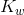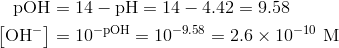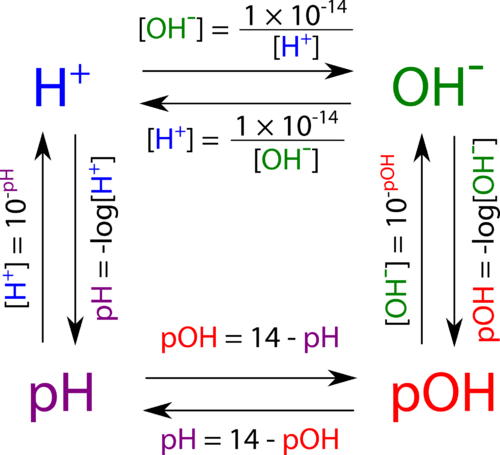## The pOH Concept

### Learning Objectives

• Define pOH.
• Perform calculations of pOH.

### Can water have healing properties?

Soap Lake has a long history as a healing place. Indian tribes would put aside their rivalries when they came to the lake to enjoy the high mineral content of the water. In the days before good antibiotics, thousands of visitors would come and enjoy the soothing waters of the lake. Soap Lake is alkaline, with water quality thought to be similar to that of the moons of the planet Jupiter.## The pOH Concept

As with the hydrogen-ion concentration, the concentration of the hydroxide ion can be expressed logarithmically by the pOH. The pOH of a solution is the negative logarithm of the hydroxide-ion concentration.

pOH = -log[OH ]

The pH of a solution can be related to the pOH. Consider a solution with a pH = 4.0. The [H + ] of the solution would be 1.0 × 10 -4  M. Dividingby this yields a [OH ] of 1.0 × 10 -10 M. Finally the pOH of the solution equals -log(1.0 × 10 -10 ) = 10. This example illustrates the following relationship.

pH + pOH = 14

The pOH scale is similar to the pH scale in that a pOH of 7 is indicative of a neutral solution. A basic solution has a pOH of less than 7, while an acidic solution has a pOH of greater than 7. The pOH is convenient to use when finding the hydroxide ion concentration from a solution with a known pH.

#### Sample Problem: Using pOH

Find the hydroxide concentration of a solution with a pH of 4.42.

Step 1: List the known values and plan the problem.

Known

• pH = 4.42
• pH + pOH = 14

Unknown

[OH-] = ? M

First, the pOH is calculated, followed by the [OH ].

Step 2: Solve.The pH is that of an acidic solution, and the resulting hydroxide-ion concentration is less than 1 × 10 -7 M. The answer has two significant figures because the given pH has two decimal places.

Figure 1 shows all of the interrelationships between [H + ], [OH ], pH, and pOH.Figure 1. Relationships between hydrogen ion concentration, hydroxide ion concentration, pH, and pOH.

### Summary

• pOH is defined.
• Calculations involving pOH are described.

### Practice

Do all calculations at the link below that involve pOH:

http://www.sciencegeek.net/APchemistry/APtaters/pHcalculations.htm

### Review

1. What is the formula for calculating pOH?
2. What pOH value is indicative of an acidic solution?
3. A pOH value of 3 would indicate what type of solution?

### Glossary

• pOH: The negative logarithm of the hydroxide-ion concentration.